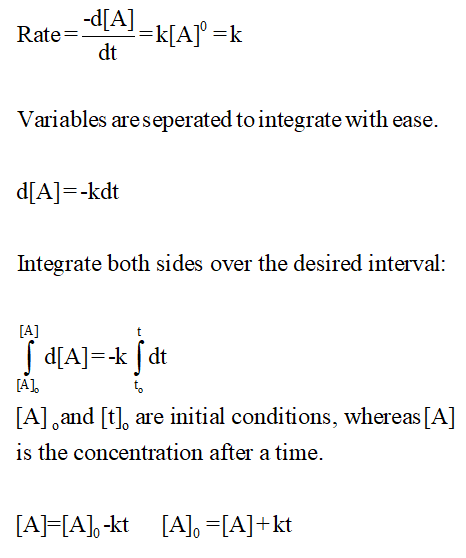# This problem is only appropriate if you have taken calculus.For a zero‑order reaction described by the chemical equationaA⟶productsthe rate expression is−dAadt=kwhere A is the reactant concentration, a is the coefficient of the reactant in the balanced chemical equation, and k is the rate constant.Derive the integrated rate expression for a zero‑order reaction. Express your answer as an algebraic formula in terms of the variables A , a , k , and t .A0=  A plot of     versus t would be linear for a zero‑order reaction.

Question
32 views

This problem is only appropriate if you have taken calculus.

For a zero‑order reaction described by the chemical equation

aA⟶products

the rate expression is

where A is the reactant concentration, a is the coefficient of the reactant in the balanced chemical equation, and k is the rate constant.

Derive the integrated rate expression for a zero‑order reaction. Express your answer as an algebraic formula in terms of the variables A , a , k , and t .

A0=

A plot of     versus t would be linear for a zero‑order reaction.
check_circle

Step 1

The differential form of the rate law of zeroth-order reaction is given by...

### Want to see the full answer?

See Solution

#### Want to see this answer and more?

Solutions are written by subject experts who are available 24/7. Questions are typically answered within 1 hour.*

See Solution
*Response times may vary by subject and question.
Tagged in